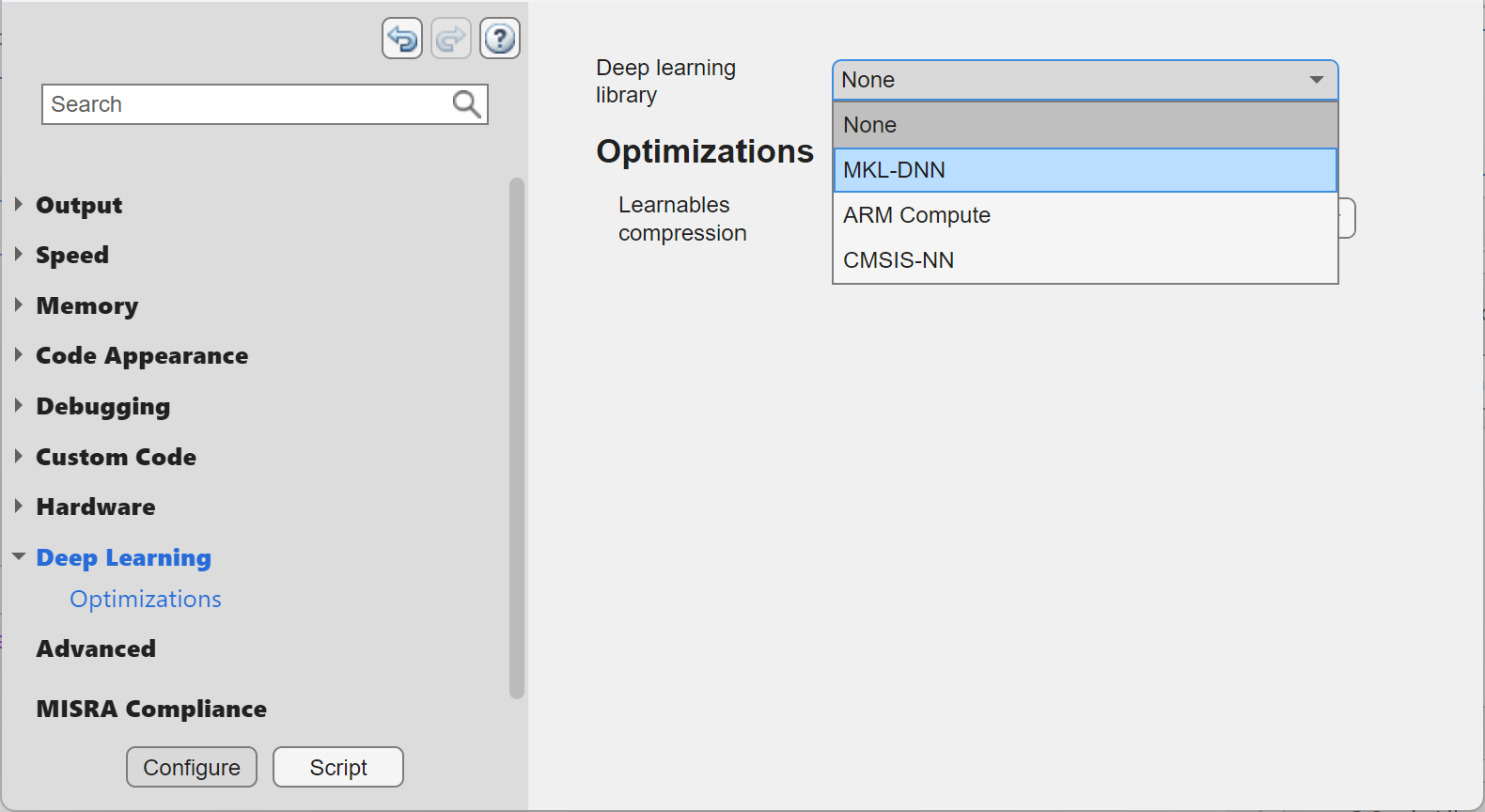## Code Generation for Deep Learning Networks with MKL-DNN

With MATLAB® Coder™, you can generate code for prediction from an already trained convolutional neural network (CNN), targeting an embedded platform that uses an Intel® processor. The code generator takes advantage of the Intel Math Kernel Library for Deep Neural Networks (MKL-DNN). The generated code implements a CNN with the architecture, layers, and parameters specified in the input `SeriesNetwork` (Deep Learning Toolbox) or `DAGNetwork` (Deep Learning Toolbox) network object.

Generate code by using one of these methods:

• The standard `codegen` command for C/C++ code generation from MATLAB code.

• The MATLAB Coder app.

### Requirements

• On Windows®, code generation for deep learning networks with the `codegen` function requires Microsoft® Visual Studio® 2015 or later.

• MATLAB Coder Interface for Deep Learning Libraries. To install this support package, select it from the MATLAB Add-Ons menu.

• Intel Math Kernel Library for Deep Neural Networks (MKL-DNN)

• Deep Learning Toolbox™.

• Environment variables for the compilers and libraries. For more information, see Prerequisites for Deep Learning with MATLAB Coder.

### Code Generation by Using `codegen`

1. Write an entry-point function in MATLAB that:

For example:

```function out = googlenet_predict(in) %#codegen % A persistent object mynet is used to load the series network object. % At the first call to this function, the persistent object is constructed and % setup. When the function is called subsequent times, the same object is reused % to call predict on inputs, thus avoiding reconstructing and reloading the % network object. persistent mynet; if isempty(mynet) mynet = coder.loadDeepLearningNetwork('googlenet'); end % pass in input out = predict(mynet,in,'MiniBatchSize',2); ```

2. Create a code generation configuration object for MEX or for a static or dynamically linked library. To specify code generation parameters for MKL-DNN, set the `DeepLearningConfig` property to a `coder.MklDNNConfig` object that you create with `coder.DeepLearningConfig`.

```cfg = coder.config('lib'); cfg.TargetLang = 'C++'; cfg.DeepLearningConfig = coder.DeepLearningConfig('mkldnn');```
3. Run the `codegen` command. Use the `-config` option to specify the configuration object. Use the `-args` option to specify the input type. The input size corresponds to the input layer size of the GoogLeNet network with `16` different images or observations.

`codegen -config cfg googlenet_predict -args {ones(224,224,3,16)} -report`

Note

You can specify half-precision inputs for code generation. However, the code generator type casts the inputs to single-precision. The Deep Learning Toolbox uses single-precision, floating-point arithmetic for all computations in MATLAB.

#### Generated Code

The network is generated as a C++ class containing an array of layer classes. The `setup()` method of the class sets up handles and allocates memory for each layer of the network object. The `predict()` method invokes prediction for each of the layers in the network. The code generator produces the function `googlenet_predict()` in `googlenet_predict.cpp` that corresponds to the MATLAB entry-point function. This function constructs the static object for the network and invokes the setup and predict methods.

Binary files are exported for layers with parameters such as fully connected and convolution layers in the network. For example, files `cnn_googlenet_conv*_w` and `cnn_googlenet_conv*_b` correspond to weights and bias parameters for the convolution layers in the network.

### Code Generation by Using the MATLAB Coder App

1. Follow the usual steps for specifying the entry-point function and specifying input types. See Generate C Code by Using the MATLAB Coder App.

2. In the Generate Code step:

• Set Language to C++.

• Click More Settings. In the Deep Learning pane, set Target library to `MKL-DNN`.3. Generate code.